×#### Thank you for registering.

One of our academic counsellors will contact you within 1 working day.

Click to Chat

1800-1023-196

+91-120-4616500

CART 0

• 0

MY CART (5)

Use Coupon: CART20 and get 20% off on all online Study Material

ITEM
DETAILS
MRP
DISCOUNT
FINAL PRICE
Total Price: Rs.

There are no items in this cart.
Continue Shopping• Complete JEE Main/Advanced Course and Test Series
• OFFERED PRICE: Rs. 15,900
• View Details

```Chapter 18 Symmetry Exercise 18.1

Question: 1

State the number of lines of symmetry for the following figures:

(i) An equilateral triangle

(ii) An isosceles triangle

(iii) A scalene triangle

(iv) A rectangle

(v) A rhombus

(vi) A square

(vii) A parallelogram

(ix) A regular pentagon

(x) A regular hexagon

(xi) A circle

(xii) A semi-circle

Solution:

(i) An equilateral triangle has 3 lines of symmetry.

(ii) An isosceles triangle has 1 line of symmetry.

(iii) A scalene triangle has no line of symmetry.

(iv) A rectangle has 2 lines of symmetry.

(v) A rhombus has 2 lines of symmetry.

(vi) A square has 4 lines of symmetry.

(vii) A parallelogram has no line of symmetry.

(viii) A quadrilateral has no line of symmetry.

(ix) A regular pentagon has 5 lines of symmetry.

(x) A regular hexagon has 6 lines of symmetry.

(xi) A circle has an infinite number of lines of symmetry all along the diameters.

(xii) A semicircle has only one line of symmetry.

Question: 2

What other name can you give to the line of symmetry of

(i) An isosceles triangle?

(ii) A circle?

Solution:

(i) An isosceles triangle has only 1 line of symmetry.This line of symmetry is also known as the altitude of an isosceles triangle.

(ii) A circle has infinite lines of symmetry all along its diameters.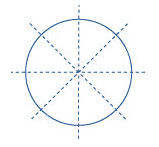Question: 3

Identify three examples of shapes with no line of symmetry.

Solution:

A scalene triangle, a parallelogram and a trapezium do not have any line of symmetry.Question: 4

Identify multiple lines of symmetry, if any, in each of the following figures:

Solution:

(A) The given figure has 3 lines of symmetry. Therefore it has multiple lines of symmetry.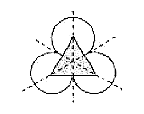(B) The given figure has 2 lines of symmetry. Therefore it has multiple lines of symmetry.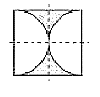(C) The given figure has 3 lines of symmetry. Therefore it has multiple lines of symmetry.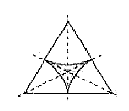(D) The given figure has 2 lines of symmetry. Therefore it has multiple lines of symmetry.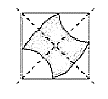(E) The given figure has 4 lines of symmetry. Therefore it has multiple lines of symmetry.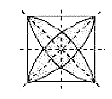(F) The given figure has only 1 line of symmetry.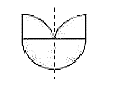(G) The given figure has 4 lines of symmetry. Therefore it has multiple lines of symmetry.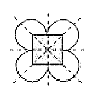(H) The given figure has 6 lines of symmetry. Therefore it has multiple lines of symmetry.```### Course Features

• 728 Video Lectures
• Revision Notes
• Previous Year Papers
• Mind Map
• Study Planner
• NCERT Solutions
• Discussion Forum
• Test paper with Video Solution